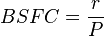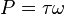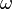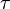## Friday, June 14, 2013

### SFOC (BSFC) Calculation With Practical Example!

How to Calculate SFOC???

Long Form of SFOC = Specific Fuel Oil Consumption
Specific fuel consumption, often shortened to SFC, is an engineering term that is used to describe the fuel efficiency of an engine design. It measures the amount of fuel needed to provide one horsepower (1hp) for a period of one hour.

Similar to SFOC - BSFC (read the difference in red text)

Long Form of BSFC = Brake Specific Fuel Consumption

Brake specific fuel consumption tell us about how much power will be produced if this much fuel is burned.
(BSFC) is a measure of the fuel efficiency of a shaft reciprocating engine. It is the rate of fuel consumption divided by the power produced. It may also be thought of as power-specific fuel consumption, for this reason.(source: wiki)
To calculate BSFC, use the formula$BSFC = \frac{r}{P}$
where:
r is the fuel consumption rate in grams per second (g/s)
P is the power produced in watts where$P = \tau \omega$$\omega$ is the engine speed in radians per second (rad/s)$\tau$ is the engine torque in newton meters (N·m)

1.Hello Frnd my frst querry ...the density which u used in sfoc calculation is at15deg cels from bdn but at the tym of injection the temperature and density of f.o will be different..the density should be taken from the graph which ll be availabe for the specific f.o which we using for the temp at the tym of injection...and my second thought the bhp for the sfoc calculation should be taken from power card for that tym and for the particular fuel...it should not be from engine manufacturer details.

RESPONSE TO CLEAR OUT THE DOUBT:

The energy contained in the fuel depends of the mass of the fuel, not on the volume, because the volume depends on temperature.
Technical fuel consumption is always measured in the laboratory by mass of fuel. This is true of wood, coal, diesel, gasoline, methane, etc. You burn so many grams or KG of fuel and get so much heat (or power) out. The reason for this is that the fuel's energy density is measured by unit weight - a gram of a particular diesel fuel makes so many calories of heat energy when burned.
But it's difficult to measure the weight of a container with a hose coming out of it, so the first thing the scientist does is take a sample of the fuel and find its density at standard temperature, and then calculate the energy density of a litre of fuel from that. It is very easy to measure the amount of ml of fuel consumed from a marked container, so this is the way it is usually done.

The power card can not be taken every time (coz if nasty c/e, he will ask you to find it every watch).So we check from the rpm that at what power does the engine is running and then take percentage of bhp..i.e, if it runs at 80% power and your engine bhp is 15600 bhp then use 80% of 15600 , i.e 12480 bhp.

source: http://listerengine.com/smf/index.php?topic=5836.0

2.it is not 31 tonnes/day but 31 metre cube /day

1.No, it has to be tonne or you can say metric tonne as if you see the formula, we are multiplying density with volume.
DENSITY=mass/volume
Thus MASS=density*volume
Most imortantly, sfoc is always calculated by mass of fuel that would produce power not volume.

3.Th author is right, fuel must be calculated in mass [kg]. On board ships fuel is usually measured in volume, I guess so they know when to fill up the tank. Burn rate is also measured on board in volume. m3/h or l/h.

It is also true that SFOC is calculated in g/kWh or kg/kWh.
Converting volume from one temperature to another is done by:

Density@15C/(1+(T-15)a)

Where T is the current temperature and a is the thermal expansion coefficient of the fuel.

1.Thank you for your valuable input!

4.Hello there

I am writing a paper regarding fuel consumption and obviously I need the SPECIFIC FUEL OIL CONSUMPTION, but I have difficulties understanding this.

Fuel consumption; 168 MT/day (heavy fuel oil) + 7.2 MT/day (marine diesel oil)
Engine power 55980 BHP

HOW TO PROCEED??????????????????????????

5.Hi all, is there a simple formula to calculate the cost contribution of the fuel (MGO & IFO180) to the final freight price: For example: previous fuel price $100, new fuel price$120, old freight per unit for a certains transport relation \$ 1500, new freight for same relation =?. I am talking about ferry boats carrying trucks (Aegean Sea) and port-port distances of ~300-400 ml. Hope it makes sense...

Have something to say? Please write here...